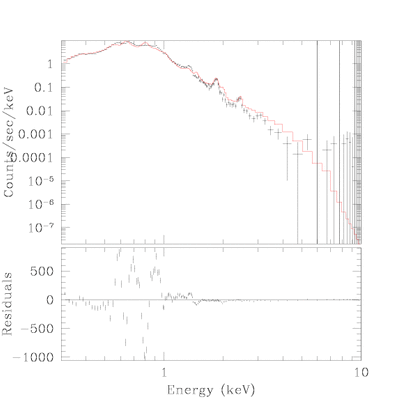True Color Image Gallery: Galactic SNR | Magellanic Cloud SNR

# Fit Detail for SNR J0505.7-6752

## Central Patch:

source=(xswabs * (xsraymond + xsraymond))
reduce chi^2 =
nh = 0.0626 10^22/cm^2
PARAMETERSDataSet 1: 134 data points -- 129 degrees of freedom.
• Statistic value = 3208.02
• Probability [Q-value] = 0
• Reduced statistic = 24.8684
--end--

## Outer Shell:

• region: Outer Shell.
• two thermal plsma model was used with abundance fixed at 0.3
source=(xswabs * (xsraymond + xsraymond))
reduce chi^2 =
nh = 0.0688 10^22/cm^2
PARAMETERSDataSet 2: 150 data points -- 145 degrees of freedom.
• Statistic value = 3299.56
• Probability [Q-value] = 0
• Reduced statistic = 22.7556
--end--

## Total:

• Above two model was merged for total specrum.
• Spectrum of three region ( Total, Central Patch, outer sheel) were simultaneously fitted with same absorption model.
• flux values in the table are from this fit result.
• Hughes et al.(1998) gives 0.04 and 0.06
source=(xswabs * ((xsraymond + xsraymond) + (xsraymond + xsraymond)))
reduce chi^2 =
nh = 0.0665 10^22/cm^2
PARAMETERSDataSet 3: 169 data points -- 160 degrees of freedom.
• Statistic value = 6780.75
• Probability [Q-value] = 0
• Reduced statistic = 42.3797
--end--

Back to SNR J0505.7-6752 IndexChandra Supernova Remnant CatalogMain Page | Galactic SNR | Magellanic Cloud SNRTrue Color Image Gallery: Galactic SNR | Magellanic Cloud SNR